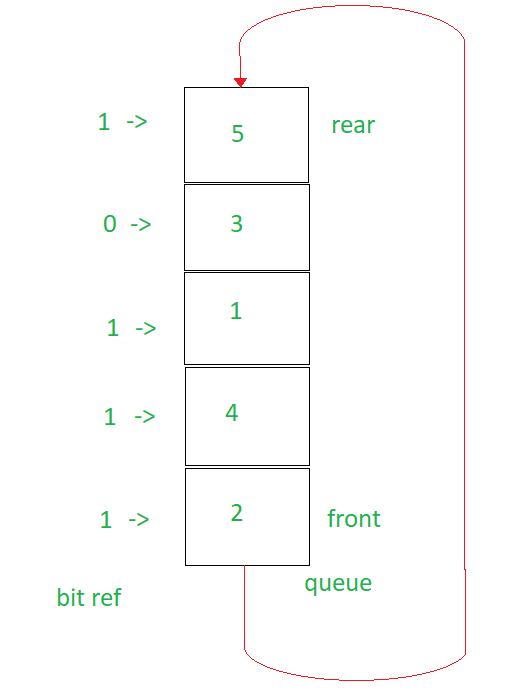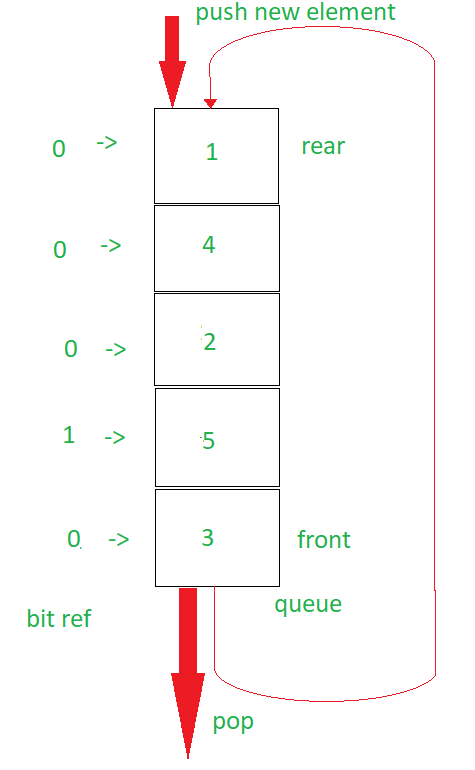# LRU Approximation (Second Chance Algorithm)

If you are not familiar with Least Recently Used Algorithm, check Least Recently Used Algorithm(Page Replacement)

This algorithm is a combination of using a queue, similar to FIFO (FIFO (Page Replacement)) alongside using an array to keep track of the bits used to give the queued page a “second chance”.

How does the algorithm work:

1. Set all the values of the bitref as False (Let it be the size of max capacity of queue).
2. Set an empty queue to have a max capacity.
3. Check if the queue is not full:
• If the element is in the queue, set its corresponding bitref = 1.
• If the element is not in the queue, then push it into the queue.
4. If the queue is full:
• Find the first element of the queue that has its bitref = 0 and if any element in the front has bitref = 1, set it to 0. Rotate the queue until you find an element with bitref = 0.
• Remove that element from the queue.
• Push the current element from the input array into the queue.

Explanation:
The bits are set as usual in this case to one for the indices in the bitref until the queue is full.
Once the queue becomes full, according to FIFO Page Replacement Algorithm, we should get rid of the front of the queue (if the element is a fault/miss). But here we don’t do that.

Instead we first check its reference bit (aka bitref) if its 0 or 1 (False or True). If it is 0 (false), we pop it from the queue and push the waiting element into the queue. But if it is 1 (true), we then set its reference bit (bitref) to 0 and move it to the back of the queue. We keep on doing this until we come across the front of the queue to have its front value’s reference bit (bitref) as 0 (false).

Then we follow the usual by removing it from the queue and pushing the waiting element into the queue.

What if the waiting element is in the queue already? We just set its reference bit (bitref) to 1 (true).We now move all the values like 2, 4, 1 to the back until we encounter 3, whose bitref is 0. While moving 2, 4, 1 to the back, we set their bitref values to 0.So now, the question how is this an approximation of LRU, when it clearly implements FIFO instead of LRU. Well, this works by giving a second chance to the front of the queue (which in FIFO‘s case would have been popped and replaced). Here, the second chance is based on the fact that if the element is seen “recently” its reference bit (bitref) is set to 1 (true). If it was not seen recently, we would not have set its reference bit (bitref) to 1 (true) and thus removed it. Hence, this is why, it is an approximation and not LRU nor FIFO.

Below is the implementation of the above approach:

 `// C++ implementation of the approach ` `#include ` `using` `namespace` `std; ` ` `  `// Function to find an element in the queue as ` `// std::find does not work for a queue ` `bool` `findQueue(queue<``int``> q, ``int` `x) ` `{ ` `    ``while` `(!q.empty()) { ` `        ``if` `(x == q.front()) ` `            ``return` `true``; ` `        ``q.pop(); ` `    ``} ` ` `  `    ``// Element not found ` `    ``return` `false``; ` `} ` ` `  `// Function to implement LRU Approximation ` `void` `LRU_Approximation(vector<``int``> t, ``int` `capacity) ` `{ ` `    ``int` `n = t.size(); ` `    ``queue<``int``> q; ` ` `  `    ``// Capacity is the size of the queue ` `    ``// hits is number of times page was ` `    ``// found in cache and faults is the number ` `    ``// of times the page was not found in the cache ` `    ``int` `hits = 0, faults = 0; ` ` `  `    ``// Array to keep track of bits set when a ` `    ``// certain value is already in the queue ` `    ``// Set bit --> 1, if its a hit ` `    ``// find the index and set bitref[index] = 1 ` `    ``// Set bit --> 0, if its a fault, and the front ` `    ``// of the queue has bitref[front] = 1, send front ` `    ``// to back and set bitref[front] = 0 ` `    ``bool` `bitref[capacity] = { ``false` `}; ` ` `  `    ``// To find the first element that does not ` `    ``// have the bitref set to true ` `    ``int` `ptr = 0; ` ` `  `    ``// To check if the queue is filled up or not ` `    ``int` `count = 0; ` `    ``for` `(``int` `i = 0; i < t.size(); i++) { ` `        ``if` `(!findQueue(q, t[i])) { ` ` `  `            ``// Queue is not filled up to capacity ` `            ``if` `(count < capacity) { ` `                ``q.push(t[i]); ` `                ``count++; ` `            ``} ` ` `  `            ``// Queue is filled up to capacity ` `            ``else` `{ ` `                ``ptr = 0; ` ` `  `                ``// Find the first value that has its ` `                ``// bit set to 0 ` `                ``while` `(!q.empty()) { ` ` `  `                    ``// If the value has bit set to 1 ` `                    ``// Set it to 0 ` `                    ``if` `(bitref[ptr % capacity]) ` `                        ``bitref[ptr % capacity] = !bitref[ptr % capacity]; ` ` `  `                    ``// Found the bit value 0 ` `                    ``else` `                        ``break``; ` `                    ``ptr++; ` `                ``} ` ` `  `                ``// If the queue was empty ` `                ``if` `(q.empty()) { ` `                    ``q.pop(); ` `                    ``q.push(t[i]); ` `                ``} ` ` `  `                ``// If queue was not empty ` `                ``else` `{ ` `                    ``int` `j = 0; ` ` `  `                    ``// Rotate the queue and set the front's ` `                    ``// bit value to 0 until the value where ` `                    ``// the bitref = 0 ` `                    ``while` `(j < (ptr % capacity)) { ` `                        ``int` `t1 = q.front(); ` `                        ``q.pop(); ` `                        ``q.push(t1); ` `                        ``bool` `temp = bitref; ` ` `  `                        ``// Rotate the bitref array ` `                        ``for` `(``int` `counter = 0; counter < capacity - 1; counter++) ` `                            ``bitref[counter] = bitref[counter + 1]; ` `                        ``bitref[capacity - 1] = temp; ` `                        ``j++; ` `                    ``} ` ` `  `                    ``// Remove front element ` `                    ``// (the element with the bitref = 0) ` `                    ``q.pop(); ` ` `  `                    ``// Push the element from the ` `                    ``// page array (next input) ` `                    ``q.push(t[i]); ` `                ``} ` `            ``} ` `            ``faults++; ` `        ``} ` ` `  `        ``// If the input for the iteration was a hit ` `        ``else` `{ ` `            ``queue<``int``> temp = q; ` `            ``int` `counter = 0; ` `            ``while` `(!q.empty()) { ` `                ``if` `(q.front() == t[i]) ` `                    ``bitref[counter] = ``true``; ` `                ``counter++; ` `                ``q.pop(); ` `            ``} ` `            ``q = temp; ` `            ``hits++; ` `        ``} ` `    ``} ` `    ``cout << ``"Hits: "` `<< hits << ``"\nFaults: "` `<< faults << ``'\n'``; ` `} ` ` `  `// Driver code ` `int` `main() ` `{ ` `    ``vector<``int``> t = { 2, 3, 2, 1, 5, 2, 4, 5, 3, 2, 5, 2 }; ` `    ``int` `capacity = 4; ` `    ``LRU_Approximation(t, capacity); ` ` `  `    ``return` `0; ` `} `

Output:

```Hits: 6
Faults: 6
```

My Personal Notes arrow_drop_upCheck out this Author's contributed articles.

If you like GeeksforGeeks and would like to contribute, you can also write an article using contribute.geeksforgeeks.org or mail your article to contribute@geeksforgeeks.org. See your article appearing on the GeeksforGeeks main page and help other Geeks.

Please Improve this article if you find anything incorrect by clicking on the "Improve Article" button below.